Electron. J. Differential Equations, Vol. 2018 (2018), No. 27, pp. 1-15.

### Composition and convolution theorems for mu-Stepanov pseudo almost periodic functions and applications to fractional integro-differential equations Edgardo Alvarez

Abstract:
In this article we establish new convolution and composition theorems for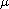-Stepanov pseudo almost periodic functions. We prove that the space of vector-valued mu-Stepanov pseudo almost periodic functions is a Banach space. As an application, we prove the existence and uniqueness of mu-pseudo almost periodic mild solutions for the fractional integro-differential equation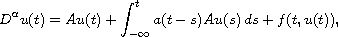where A generates an-resolvent family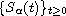on a Banach space X,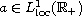,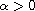, the fractional derivative is understood in the sense of Weyl and the nonlinearity f is a mu-Stepanov pseudo almost periodic function.

Submitted September 12, 2016. Published January 18, 2018.
Math Subject Classifications: 45D05, 34A12, 45N05.
Key Words: mu-Stepanov pseudo almost periodic; mild solutions,; fractional integro-differential equations; composition; convolution.

Show me the PDF file (268 KB), TEX file for this article.Edgardo Alvarez Universidad del Norte Departamento de Matemáticas y Estadística Barranquilla, Colombia email: edgalp@yahoo.com, ealvareze@uninorte.edu.co